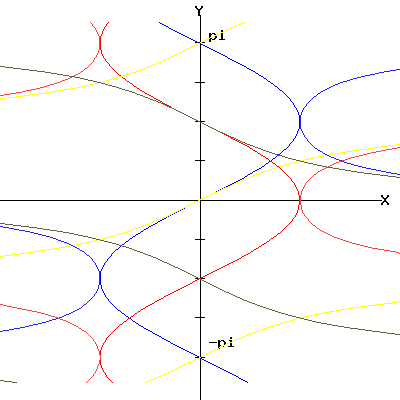Egwald Mathematics: Trigonometry

by

Elmer G. Wiens

Egwald's popular web pages are provided without cost to users.
Please show your support by joining Egwald Web Services as a Facebook Fan:Follow Elmer Wiens on Twitter:Inverse Natural Trigonometric Functions

The natural trigonometric functions, like sine and cosine, yield a number from an angle. Thus, for z = sin(alpha), if the angle alpha = pi/6, then .5 = sin(alpha). Suppose, now, we want to find the angle, alpha, such that sin(alpha) = z = 1. Then alpha = pi/2, since sin(pi/2) = 1. Once we know alpha, we can find the values of x and y that produce the angle alpha on the circle on the natural trig page functions.

Since we can do this for any angle, we can define a new function called the inverse sine or "arcsin" function by alpha = arcsin(z). For convenience, let us re-label the variables alpha and z, by y and x. (Don't confuse these new y and x labels with the ones on the circle that locate the point P).

Furthermore, we can define inverse functions for each of the natural trigonometric functions: arcsin, arcos, arctan, arccse, arcsec, and arccot.

 arcsin arccos arctan arccsc arcsec arccotCheck the option you want Graph natural inverse functions Graph reciprocal inverse functions Graph inverse natural and reciprocal trigonometric functionsCopyright © Elmer G. Wiens:   EgwaldWeb ServicesAll Rights Reserved.    Inquiries